## Solar Cell

CASPOC provides the solar cell library so that users can add solar panels into their simulation. The simplest use is to take a solar cell and its corresponding sunlight setting. In an electrical circuit, solar cell is a DC voltage source. We can simply use the electrical load to see its voltage and current characteristics as below.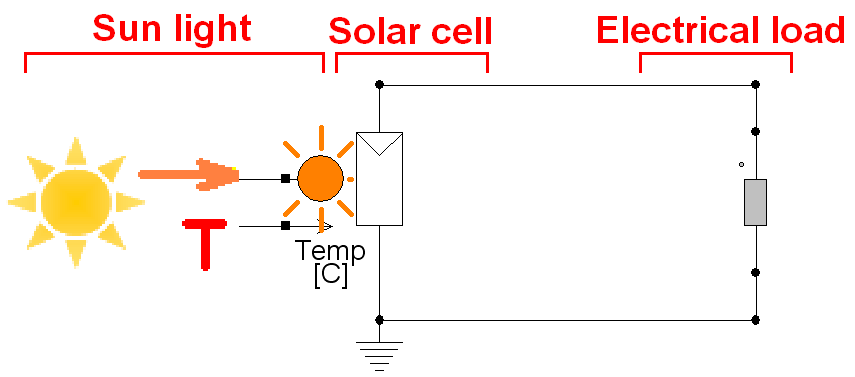### Solar cell

Left-click Components/Library/GreenEnergy/Solar/SolarCell (step 1) and release the mouse. The solar cell follows the cursor and right-click to change its direction if needed (step 2). Left-click to put it on the workscreen and right-click on it to change its parameters (step 3) if needed. In the pop-window, we can see the parameters as below:

• Isc = 5 (short circuit current [A] per 1000 Watt/m�)
• Rparallel = 100k ohm (parallel resistance of the solar cell)
• Rseries = 10 m ohm (series resistance of the solar cell)
• Tnominal = 25 (nominal temperature for which Voc and Isc are defined)
• Voc = 35 volt (open circuit voltage)
• Alpha[A/Celsius] = 2.06m (temperature dependency of the short circuit current Isc)
• Alpha[V/Celsius] = -0.152 (temperature dependency of the open circuit voltage Voc)Then use a resistor as an electrical load for the solar cell. The goal of the resistor is to get the voltage and current value of the circuit. Hence we need voltage and current sensors around the resistor. Alternatively, we can also use another resistor model which includes a voltage sensor and a current sensor as below. The component is named variable load. The only difference between the two models is that a resistor provides a fixed resistance, and a variable load can provide either a fixed or a variable resistance.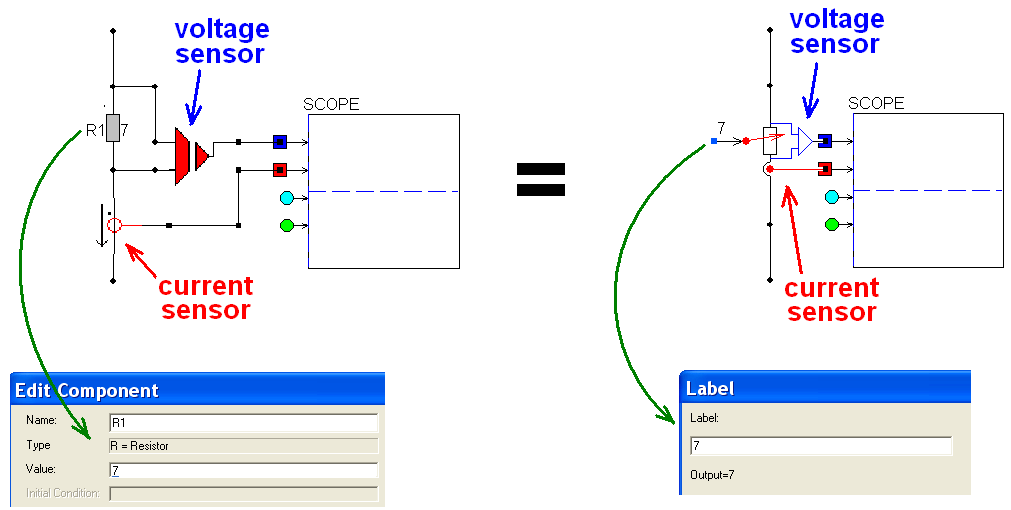Left-click Components/Library/GreenEnergy/Solar/VariableLoad (step 1) and release the mouse. Left-click on the workscreen to put the variable load in the right side of the solar cell. Right-click its left square dot to enter the resistance value (step 2). In the pop-window, enter label = 7 (step 3) and click OK to save the setting (step 4).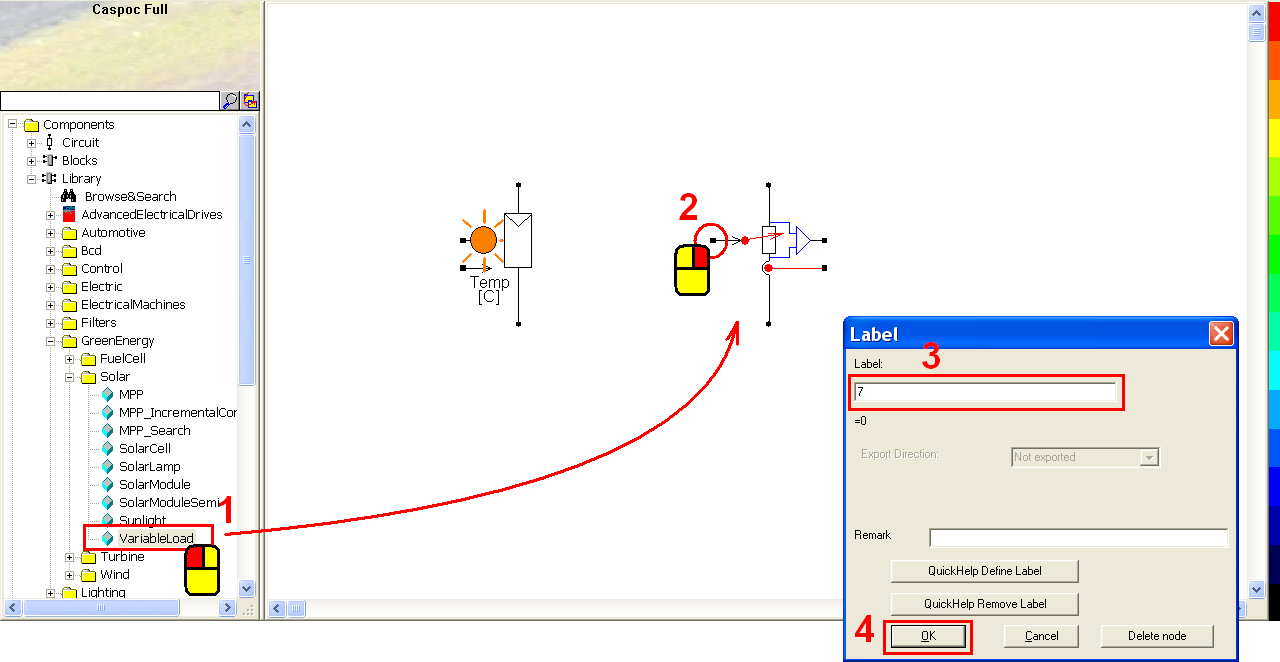Connect the solar cell and the variable load with the following configuration (step 1). Currently we have not set an electric ground. Right-click on the cathode of solar cell (step 2) to add a ground label. In the pop-window, enter Label = Ground (step 3) and click OK to save the setting (step 4).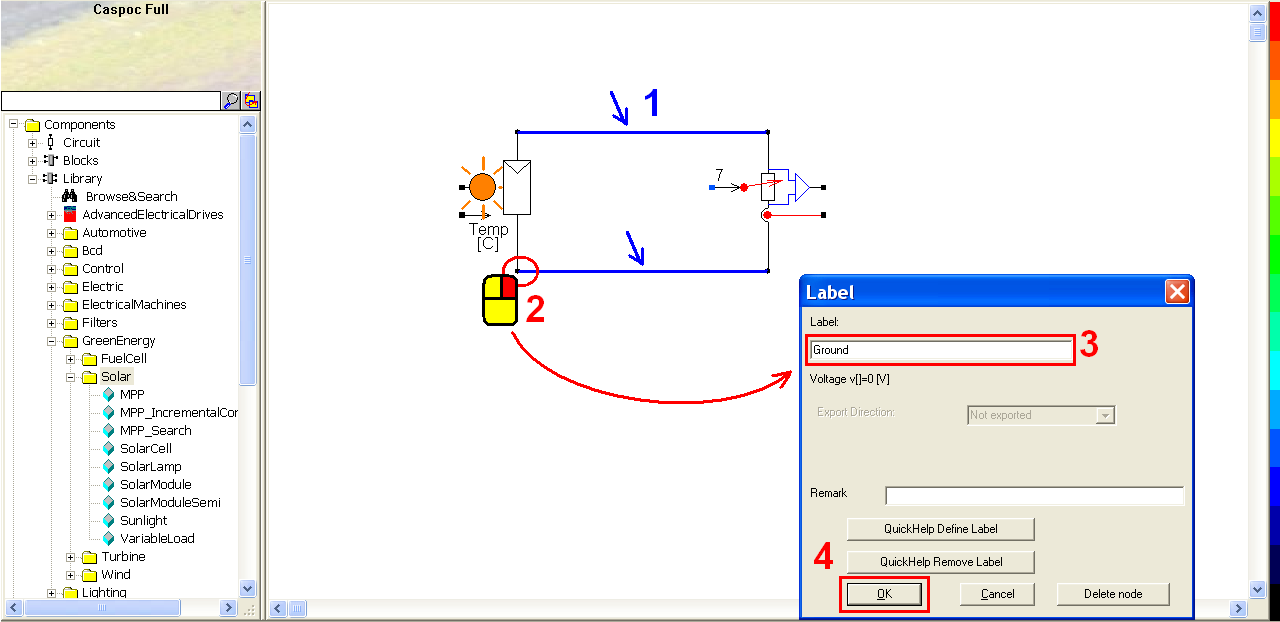Before running the simulation, we shall set the natural conditions for the solar panel, namely the sunlight density and the temperature. Left-click Components/Library/GreenEnergy/Solar/Sunlight (step 1) and release the mouse. Right-click the sunlight (step 2) to modify its parameters if needed. In the pop-window, we can see the parameters:

• Sunlight Density [watt/mm�] = 1000 (sunlight density at the normalized distance)
• Temperature [Celsius] = 25 (temperature of the solar cells in Celsius)
• Angle [degrees] = 0 (incidence angle of the sunlight)
• Extra Distance [m] = 0 (extra distance)

Click OK to save the setting (step 4).Now we can start to run a simulation. Click the scope iconin the experience bar (step 1) to put a scope on the workscreen. Left-click the right-bottom corner of the scope and hold down the mouse to enlarge this scope (step 2).Left-click the scope and hold down the mouse to move it to receive the two square dots of the variable load (step 1). Make sure that the first two input traces (in blue and in red) become a square as below. Then click the short-cut of simulation parameter(step 2). In the pop-window, select Euler for the Numerical Integration Method (step 3), Tscreen = 1, dt = 100u (step 4) and then click OK to save the setting (step 5).Click the short-cut of start simulation(step 1). Right-click on the scope (step 2) to see the details of the simulation result. Click the shortcut of listbox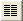(step 3) to enable the numeric display of simulation result (step 4).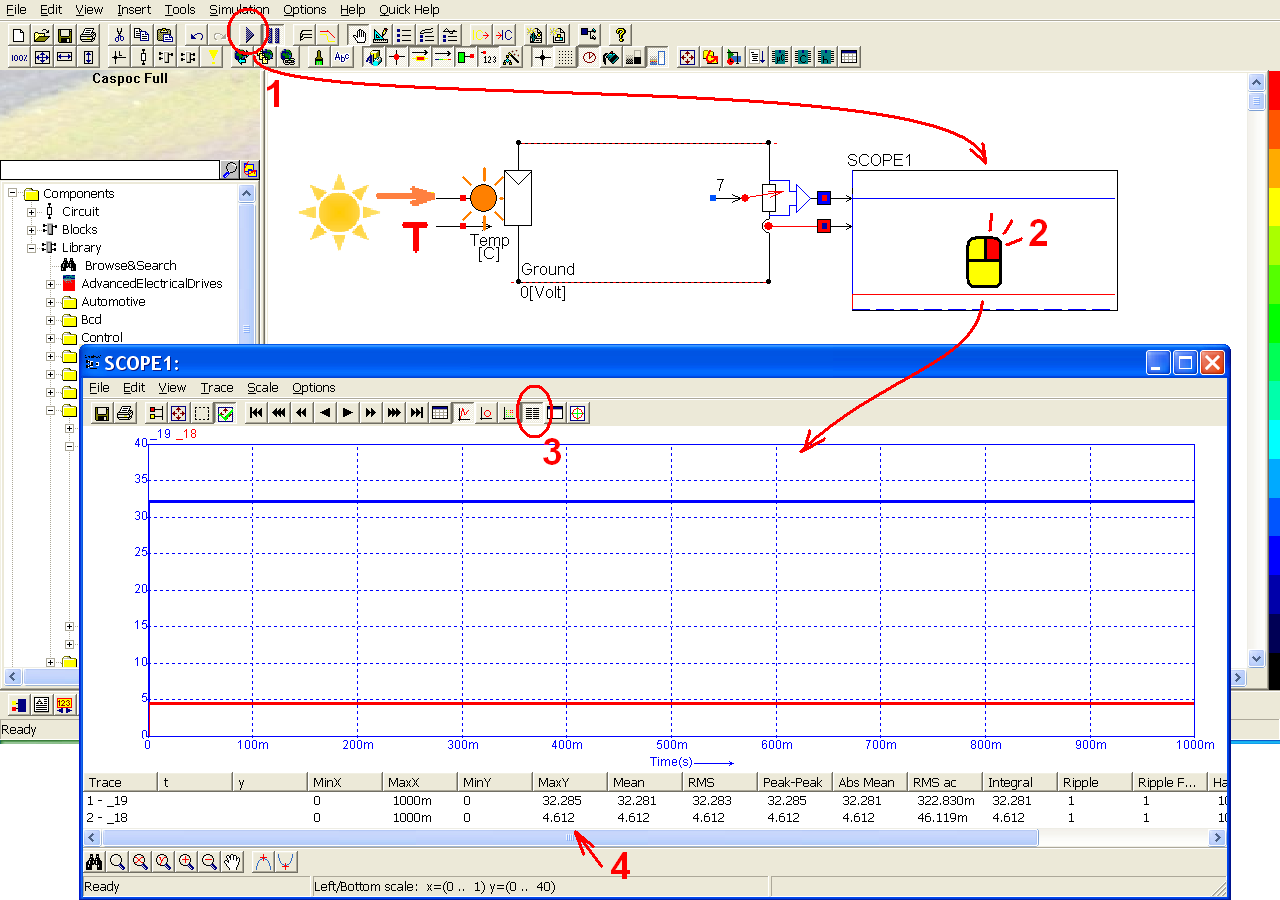### Solar cells in series

Left-click the connection between the cathodes of the solar cell and the variable load (step 1). Click the Delete key to delete this connection (step 2). Right-click the cathode of the solar cell to remove the ground label (step 3). In the pop-window, enter label = blank (step 4) and click OK to save the setting (step 5).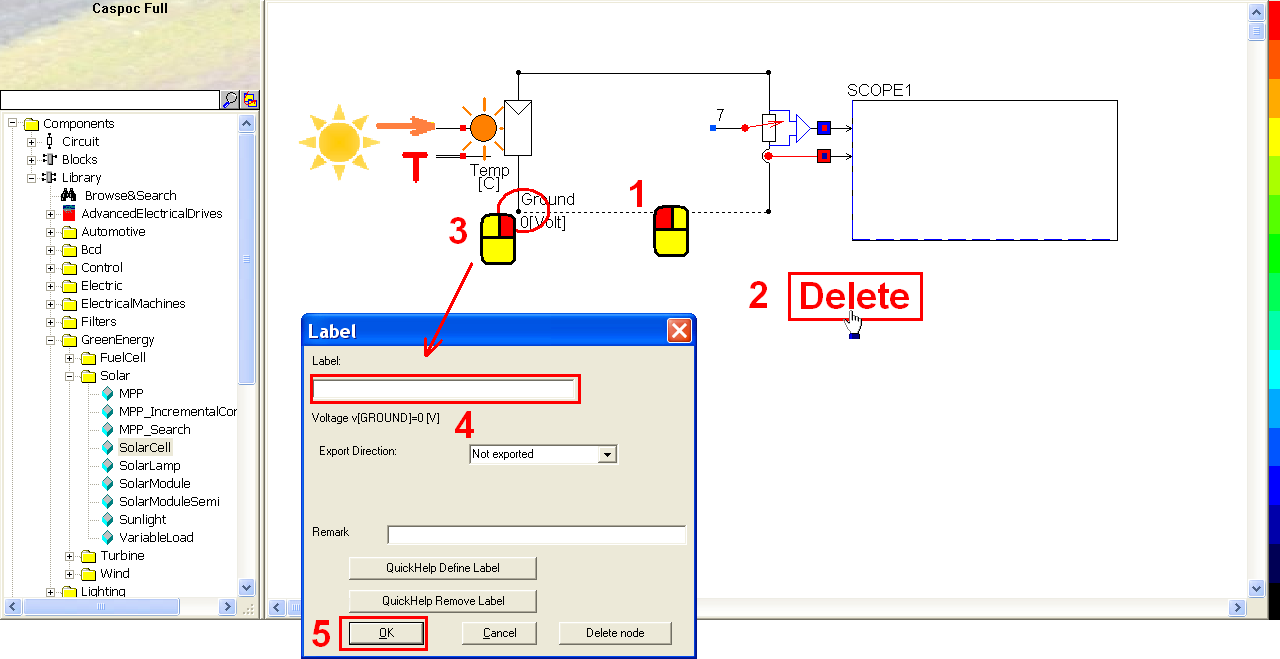Left-click Components/Library/GreenEnergy/Solar/SolarCell (step 1) and release the mouse. Left-click on the workscreen to put one more solar cell below the first solar cell (step 2). Repeat the same steps to put two more solar cells (step 3 and step 4).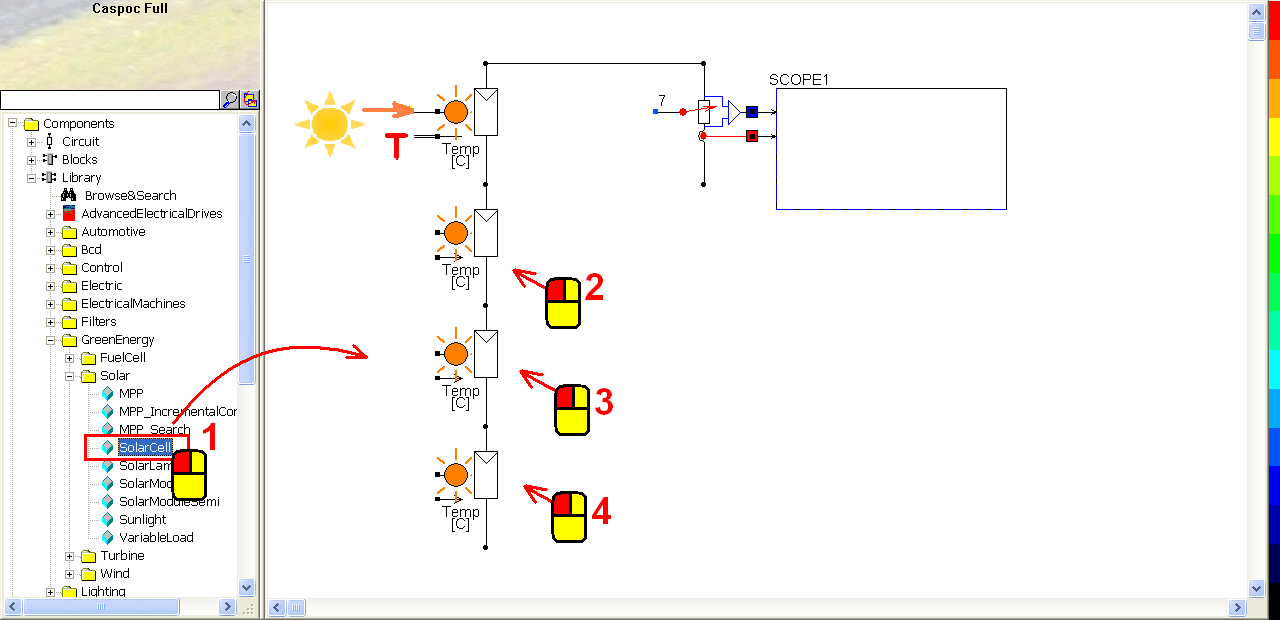Similarly, left-click Components/Library/GreenEnergy/Solar/Sunlight (step 1) and release the mouse. Re-click on the workscreen to put three more sunlight components for the three solar cells (step 2).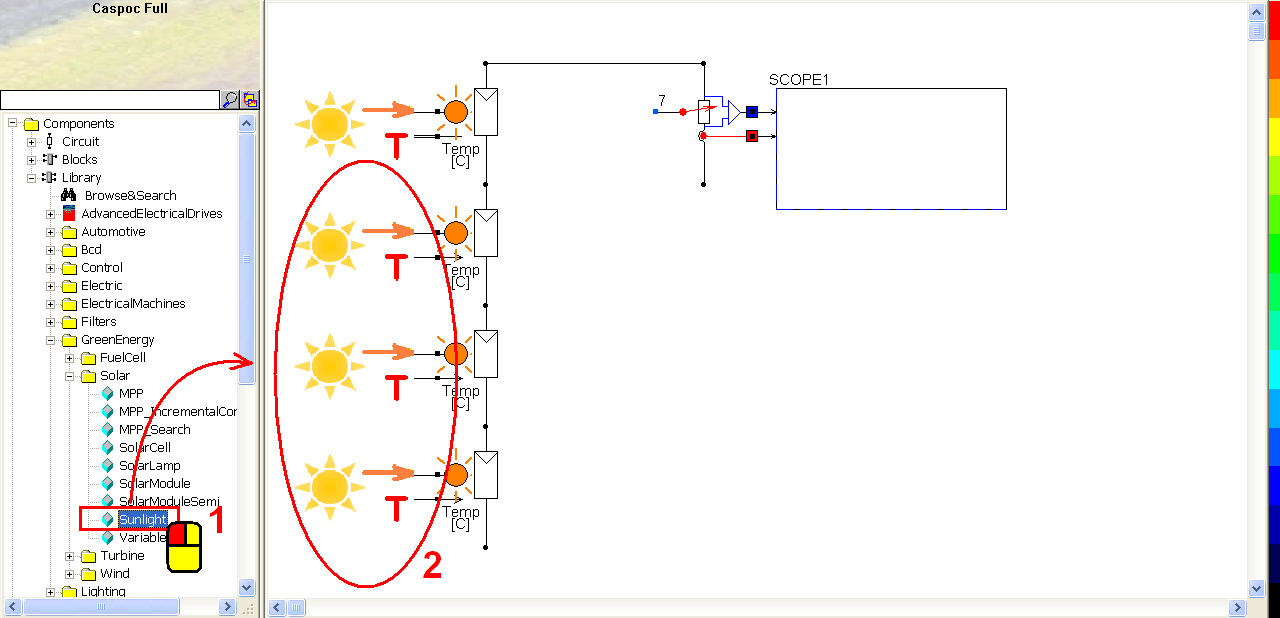Connect the cathode of the fourth solar cell and the variable load with the following configuration (step 1). We have not set an electrical ground so far. Right-click the cathode of the fourth solar cell (step 2) to add a ground label. In the pop-window, enter label = ground (step 3) and click OK to save the setting (step 4).Because of the four solar cells in series, the voltage value is multiplied by 4. Right-click the left square dot the value (step 1) to change the resistance. In the pop-window, enter label = 28 (step 2) and click OK to save the setting (step 3).Click the short-cut of start simulation(step 1). Right-click on the scope (step 2) to see the details of the simulation result. Click the shortcut of listbox(step 3) to enable the numeric display of simulation result (step 4).### Solar cells in parallel

Left-click the connection between the cathodes of the fourth solar cell and the variable load (step 1). Click the Delete key to delete this connection (step 2).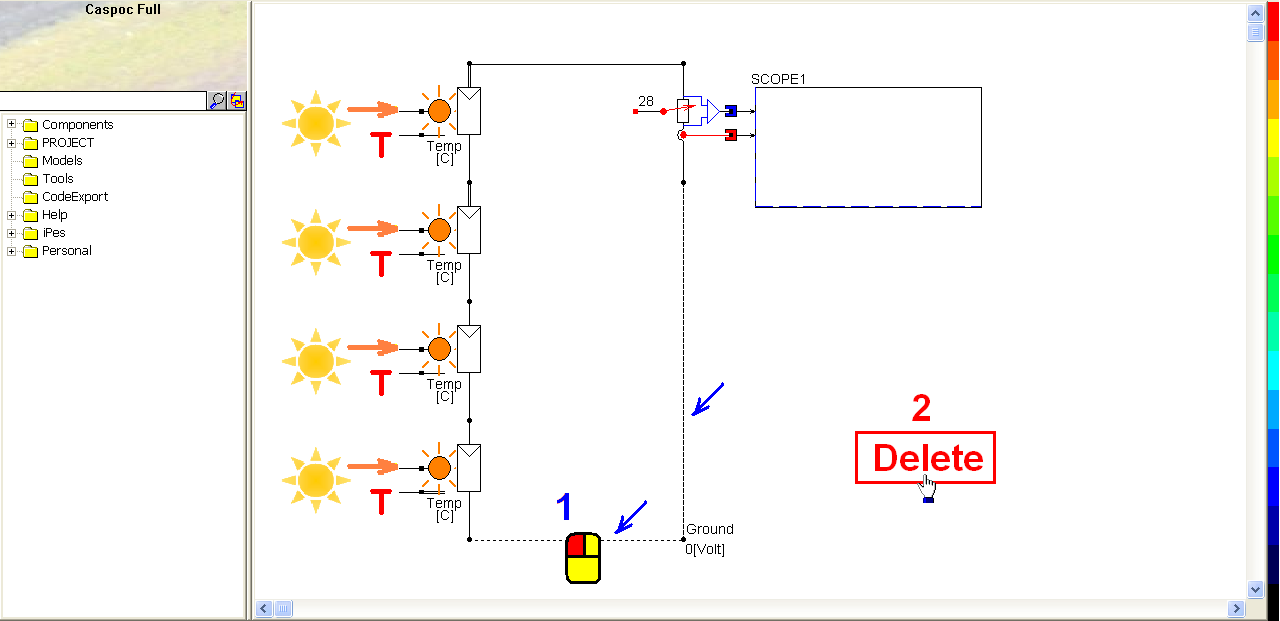Before changing the configuration of the solar cells from series to parallel, we shall add a ground label which is deleted in the previous step. In this way, we don�t get any suggestion message in each step. Right-click the cathode of the second solar cell (step 1) to add a ground label. In the pop-window, enter label = Ground (step 2) and click OK to save the setting (step 3). Left-click and draw the mouse to select the variable load and the scope (step 4). Release the mouse after the selection. Left-click again on the selection area and draw to move them to the right side (step 5).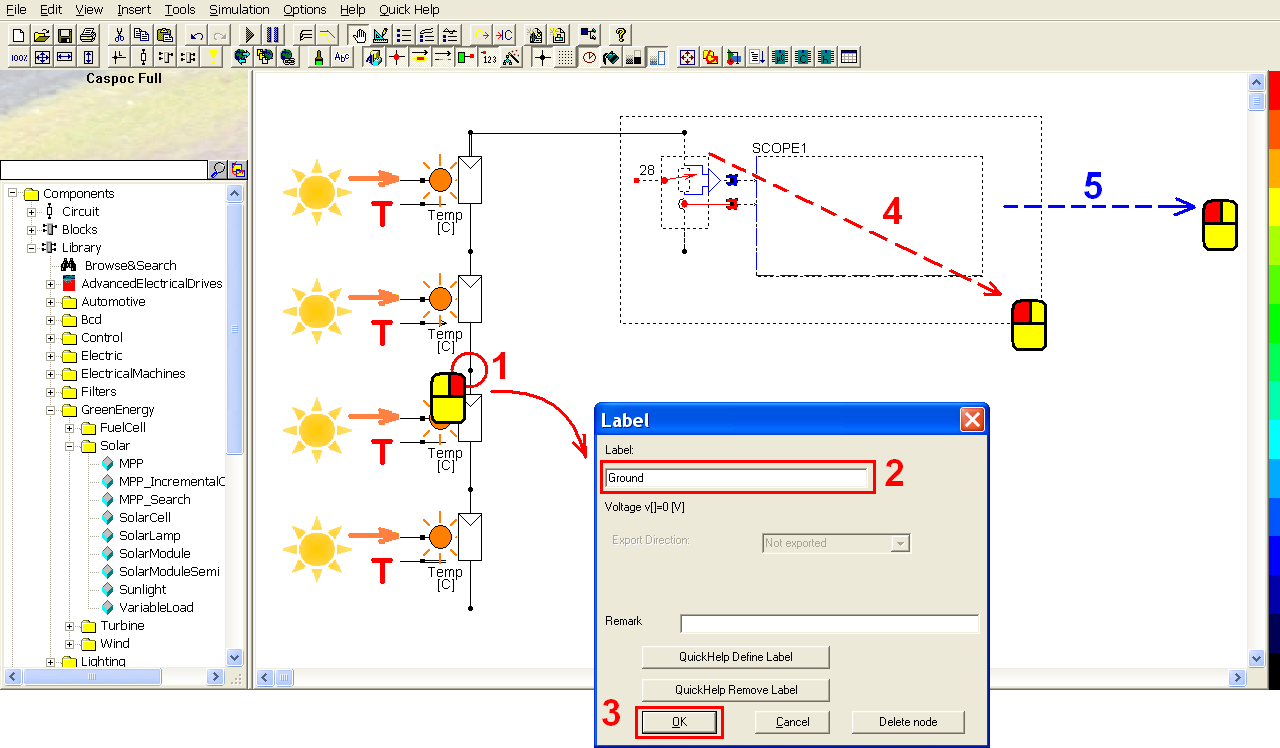Left-click the third solar cell and hold down the mouse to move it to the left side of the variable load.Similarly, select the remaining components and move them to the left side of the variable (step 1). In this way, the two solar cells will be across to the other two solar cells as below. Connect these solar cells and the variable load with the following configuration (step 2).Right-click on the left square dot of the variable load (step 1) to change its resistance. Enter label = 7 (step 2) and click OK to save the setting (step 3).Click the short-cut of start simulation(step 1). Right-click on the scope (step 2) to see the details of the simulation result. Click the shortcut of listbox(step 3) to enable the numeric display of simulation result (step 4).### Characteristic curve of solar cell

Left-click and draw to select the two solar cells in the right side and the variable load (step 1). Click the Delete key (step 2) to delete them. Similarly, select one of the remaining solar cells (step 3) and click the Delete key to delete it. Make sure there is only one solar cell with a ground label on the workscreen.Left-click Components/Circuit/RLC/C (step 1) and release the mouse. The capacitor follows the cursor and right-click to change its direction (step 2). Until the anode of the capacitor is upward, left-click on the workscreen to put the capacitor in the right side of the solar cell. Right-click on the capacitor (step 3) to modify its parameter. In the pop-window, enter value = 1 (step 4) and click OK to save the setting (step 5).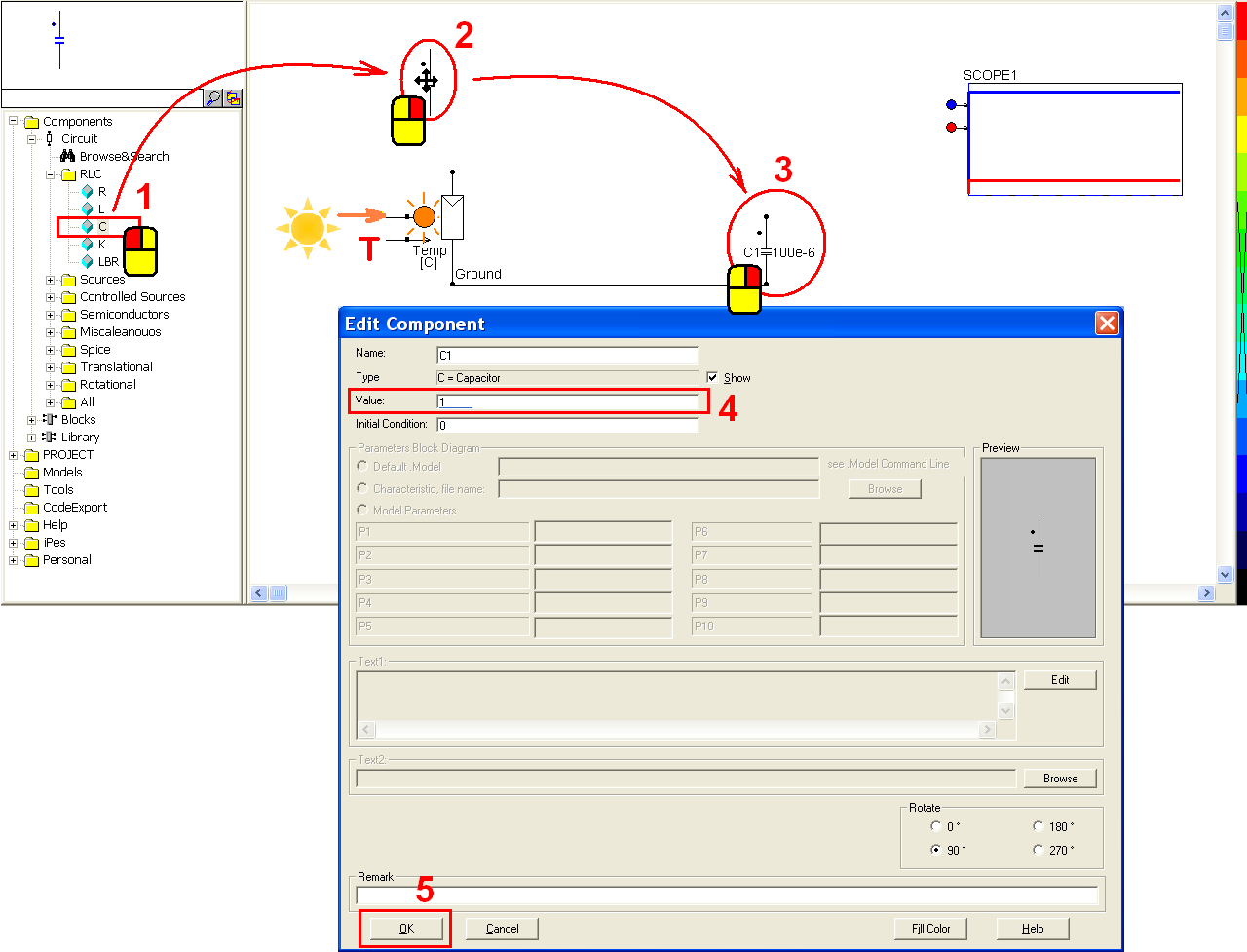Left-click the first scope and hold down to move it to be closer to the capacitor (step 1). Connect the anode of the capacitor to the first blue input trace (step 2). Right-click the second red input trace (step 3) to get the current value of the capacitor C1. In the pop-window, enter Trace=Current through: C1 (step 4) and click OK to save the setting (step 5).Right-click the scope (step 1) to open the scope window. In the scope window, select View/XY Writer (step 2). Then close this window.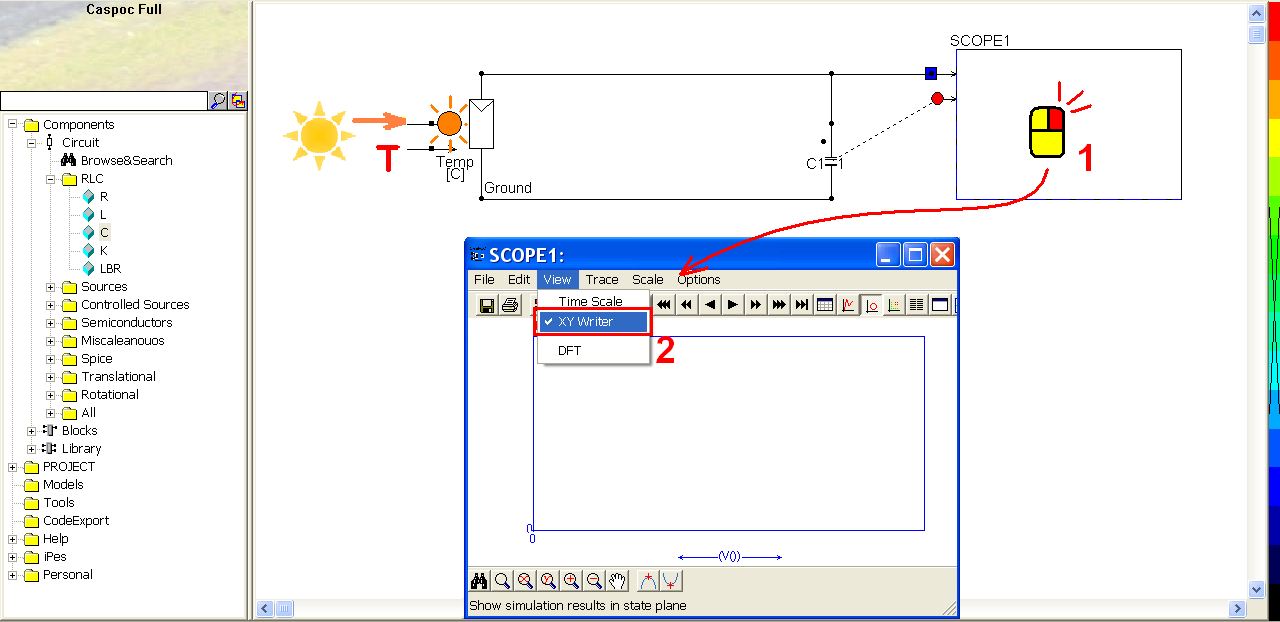Click the short-cut of simulation parameter(step 1). In the pop-window, select Euler for the Numerical Integration Method (step 2), Tscreen = 10, dt = 1mu (step 3) and then click OK to save the setting (step 4).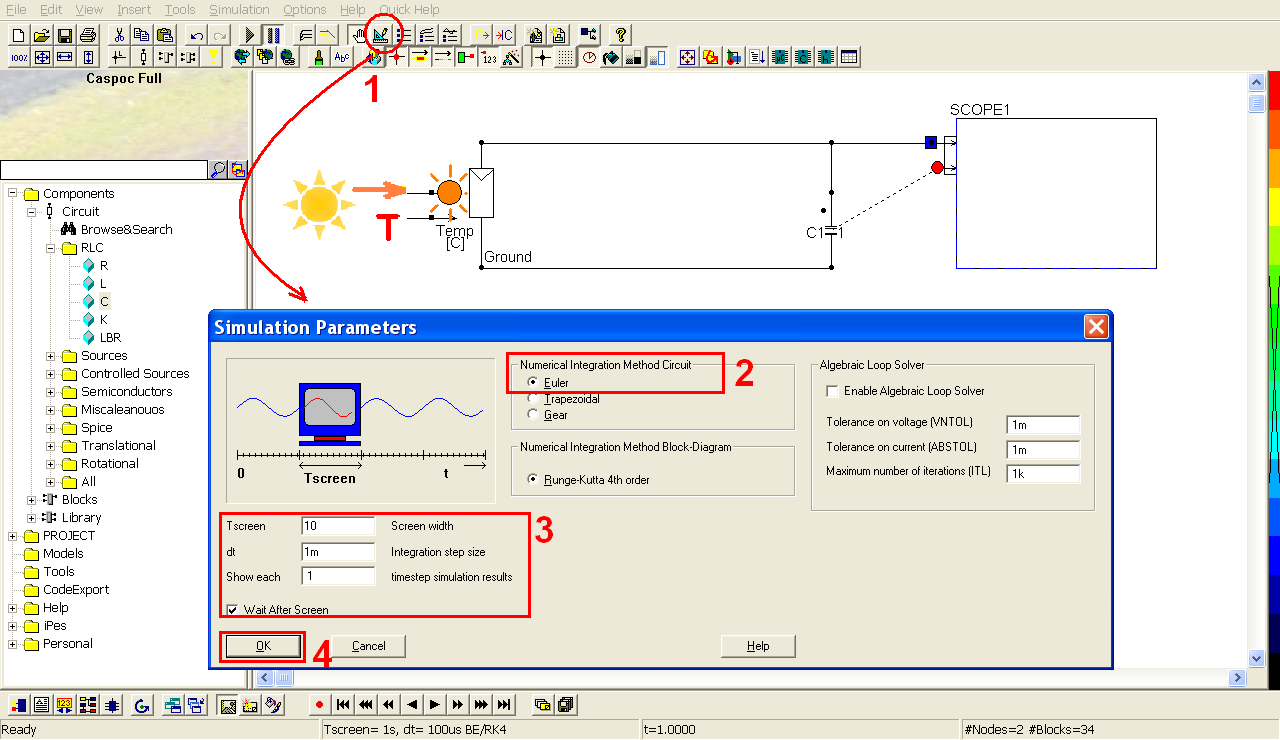Click the short-cut of start simulation(step 1). Right-click on the scope (step 2) to see the details of the simulation result (step 3).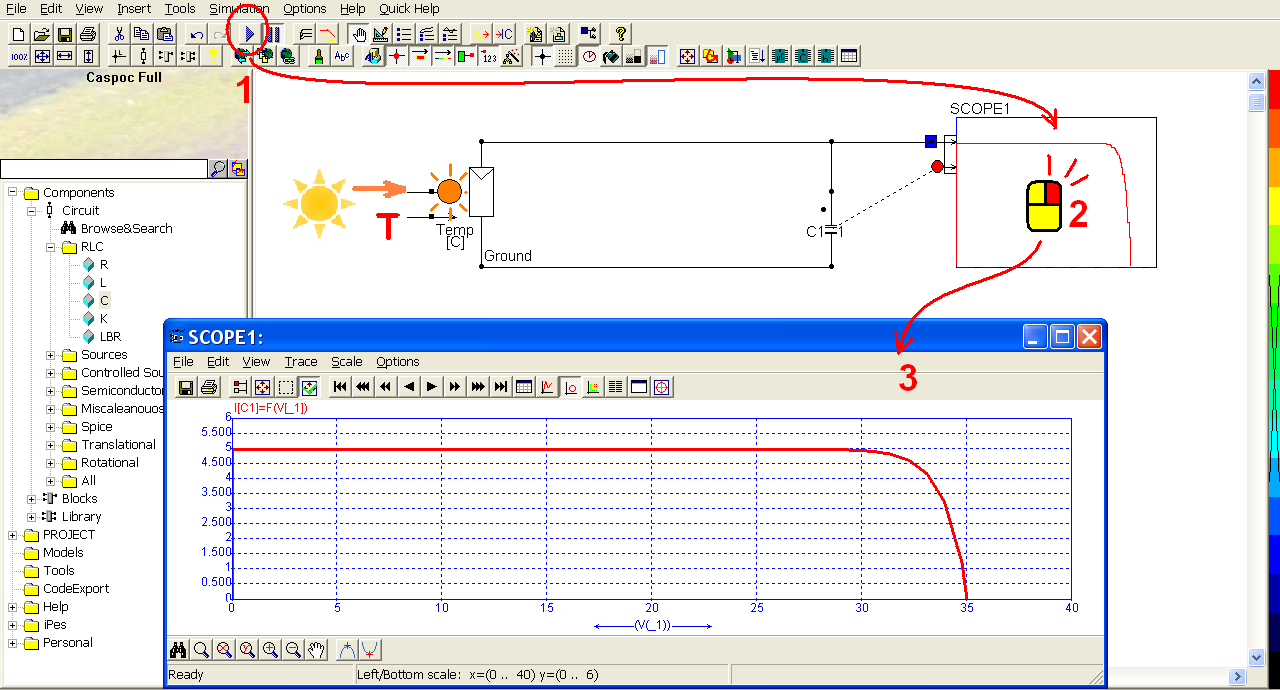### Maximum power point tracking

Click the Ctrl key and hold it down (step 1). Select all the components and connections besides the solar cell (step 2, 3, 4, 5 and 6). Release the Ctrl key and then click the Delete key on the keyboard to delete them.Check there is a ground label in the cathode of the solar cell (step 1). If not, right-click the cathode (round dot) and add it.

Left-click Components/Library/PowerConverters/Averaged/BoostAveraged (step 2) and release the mouse. The averaged boost converter follows the cursor and right-click to change its direction if needed (step 3). Until the input of the boost converter is leftward and the output is rightward, left-click on the workscreen to put it in the right side of the solar cell (step 4).Left-click Components/Library/Electric/Battery/BatterySOC (step 1) and release the mouse. The battery follows the cursor and left-click on the workscreen to put it in the right side of the boost converter. Right-click on the battery to modify its parameters (step 2). In the pop-window, click �Help Lib� (step 3) to see the details of these parameters and modify them:

• Capacity = 6.5Ah (capacity of the battery in Amperes times hour)
• Idischarge = 1.3A (constant discharge current associated with the discharge curve)
• Rinternal = 4.6m Ohm (internal resistance)
• SoC (t0) = 50% (state of charge at t=0) (step 4)
• Texp = 1 hour (end time of the first exponential decay)
• Tnom = 4 hours (time where the battery reaches the nominal voltage Vnom)
• Vexp = 325 volts (voltage at Texp) (step 5)
• Vnom = 320 volts (voltage at Tnom) (step 5)
• Voc = 340 volts (open circuit voltage) (step 5)
• Texp, Tnom, Vexp, Vnom are read from the discharge curve for a constant discharge current specified at Idischarge
• Select �Flip Vertical� (step 6) and click OK to save the setting (step 7).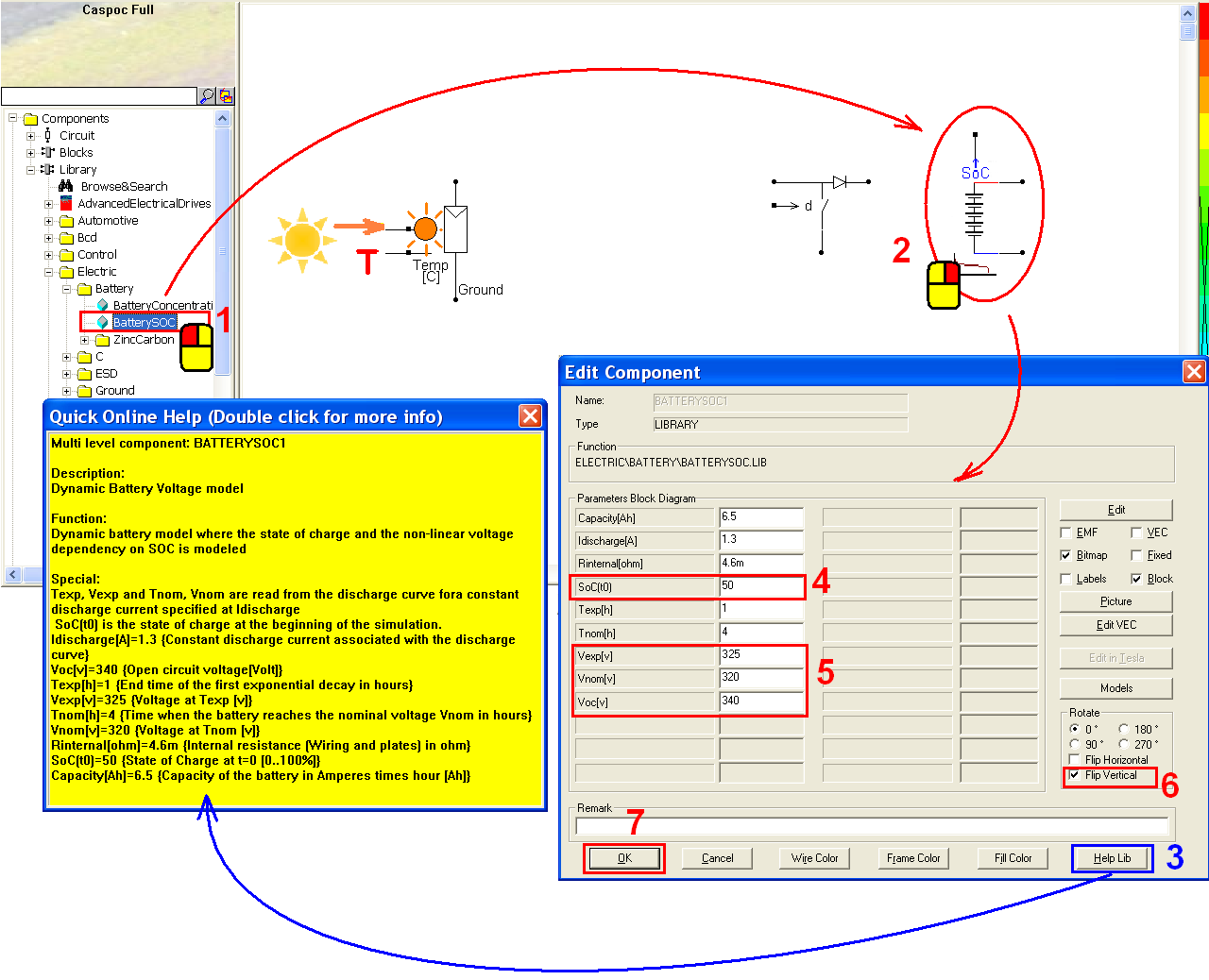Left-click to select the battery. Hold down the mouse button to move the battery in order to connect with the averaged boost converter (step 1). Connect the cathode of the battery and the switch (step 2). Draw the mouse button to select the sunlight component (step 3) and then click the Delete key (step 4) to delete it. Right-click the solar cell (step 5) to modify its parameters: Rparallel = 10k ohm, Rseries = 100m ohm, Voc = 200 volts (step 6). Click OK to save the setting (step 7).Left-click Components/Library/GreenEnergy/Solar/MPP_Search (step 1) and release the mouse. Then left-click on the workscreen to put the MPP searcher in the left-bottom corner of the boost converter. Connect the MPP searcher, the boost converter, and the solar cell with the following configuration (step 2). Right-click on the MPP searcher (step 3) to modify its parameters in the pop-window:

• Delta_d = 0.005 or 5m (incremental step size for the output duty cycle)
• d0 = 1 (initial output duty cycle) (step 4)

Click OK to save the setting (step 5).

Then we shall connect the averaged boost converter and the solar cell (step 6). Before that, we need two sensors to measure the voltage and current value for the MPP searcher (power equals to the product of the voltage and current) (step 7).Left-click Components/Library/Sensor/Current/i (step 1) and release the mouse. The current sensor follows the cursor and left-click to put it between the boost converter and the solar cell. Right-click on the current sensor (step 2) to modify the parameter: R= 1u ohm (step 3). Set the rotation to: 0 degree and Flip vertical (or 180 degree and Flip Horizontal) (step 4). Click OK to save the setting (step 5).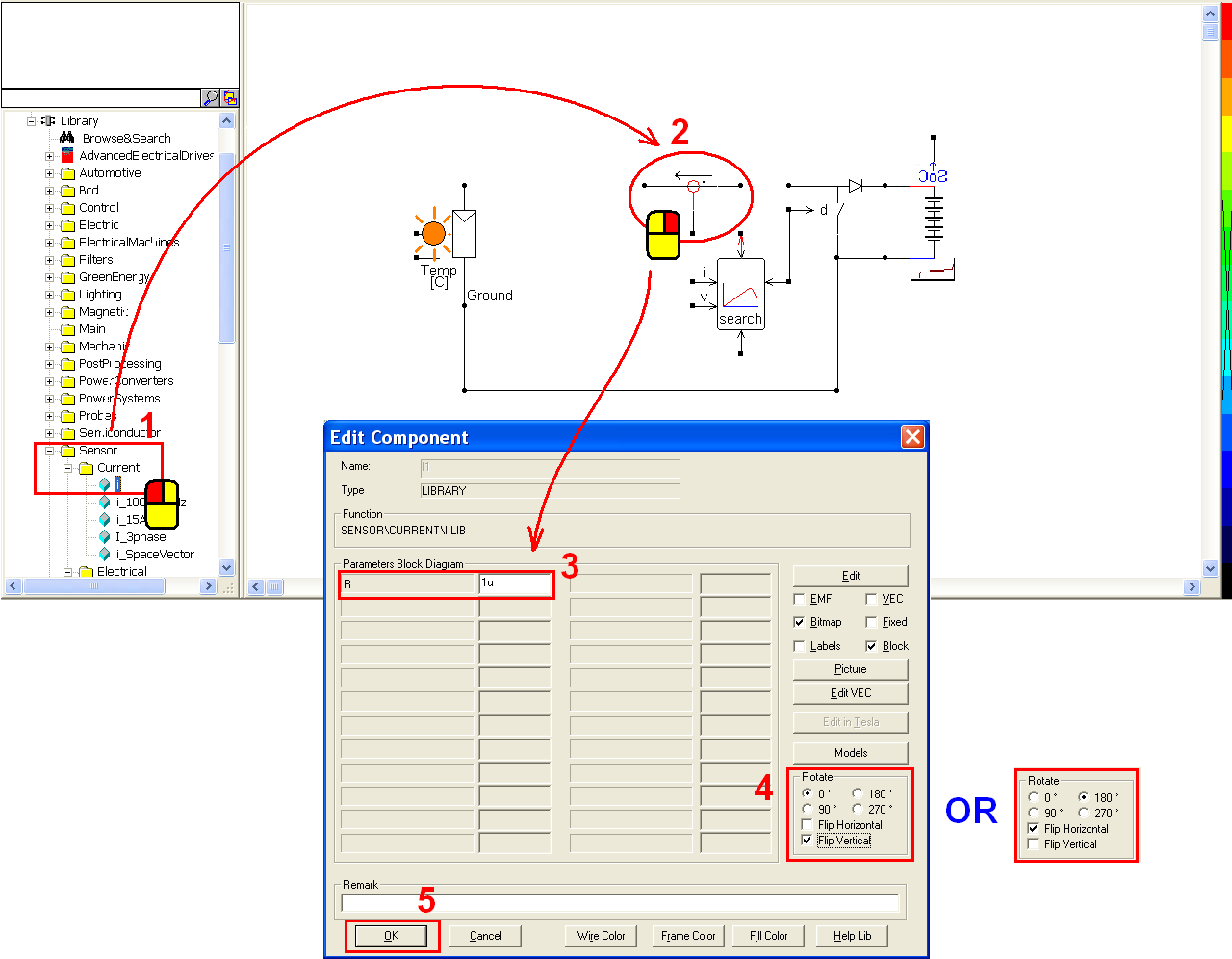Left-click Components/Library/Sensor/Voltage/v (step 1) and release the mouse. The voltage sensor follows the cursor and right-click to change its direction if needed (step 2). Until the anode of the voltage sensor is leftward, click in the left side of the MPP searcher to send its output voltage value to the input voltage value of the MPP search (step 3). Make sure that the output of the voltage sensor is well connected with the second input of the MPP searcher (step 4).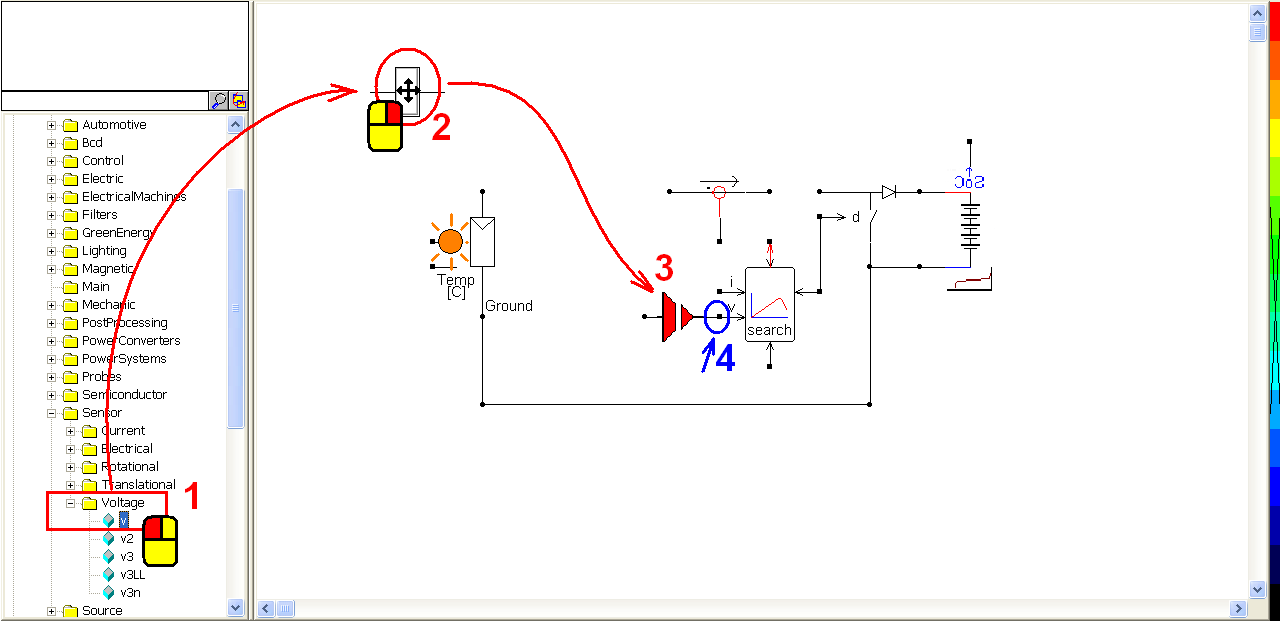Connect the current sensor, the boost converter and the solar cell with the following configuration (step 1). Connect the anode of the solar cell to the input of the voltage sensor (step 2). Click the scope iconin the experience bar (step 3) and put it above the battery to get its State of Charge (SoC). Make sure that the SOC output is connected with the first input trace of the first scope (step 4). Similarly, click the scope iconin the experience bar (step 5) and put it above the first scope. Connect the cathode of the diode in the boost converter to the first input trace of the second scope (step 6) to get the voltage value.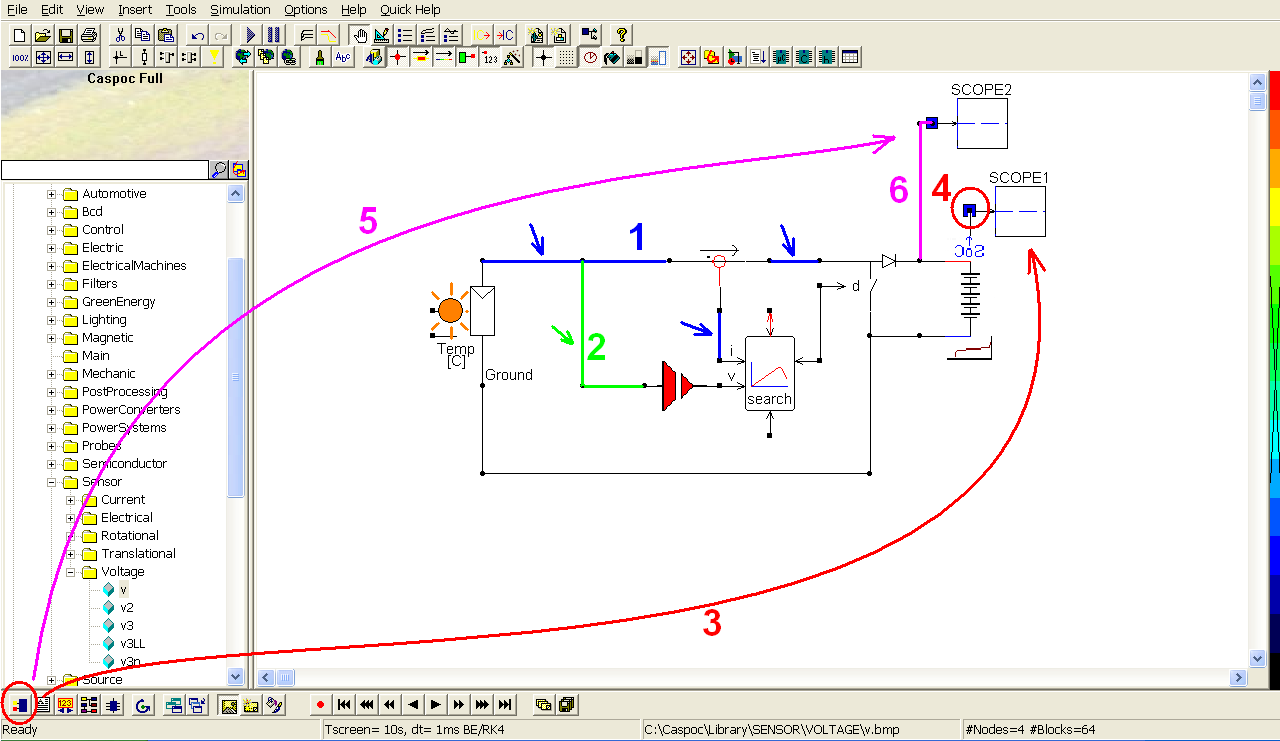Right-click the output (square dot) of the current sensor (step 1). In the pop-window, enter label = I_dc (step 2) and click OK to save the setting (step 3). Right-click the output (square dot) of the voltage sensor (step 4). In the pop-window, enter label = V_dc (step 5) and click OK to save the setting (step 6). Right-click the upward power output of the MPP searcher (step 7). Enter label = P_solar in the pop-window (step 8) and click OK to save the setting (step 9).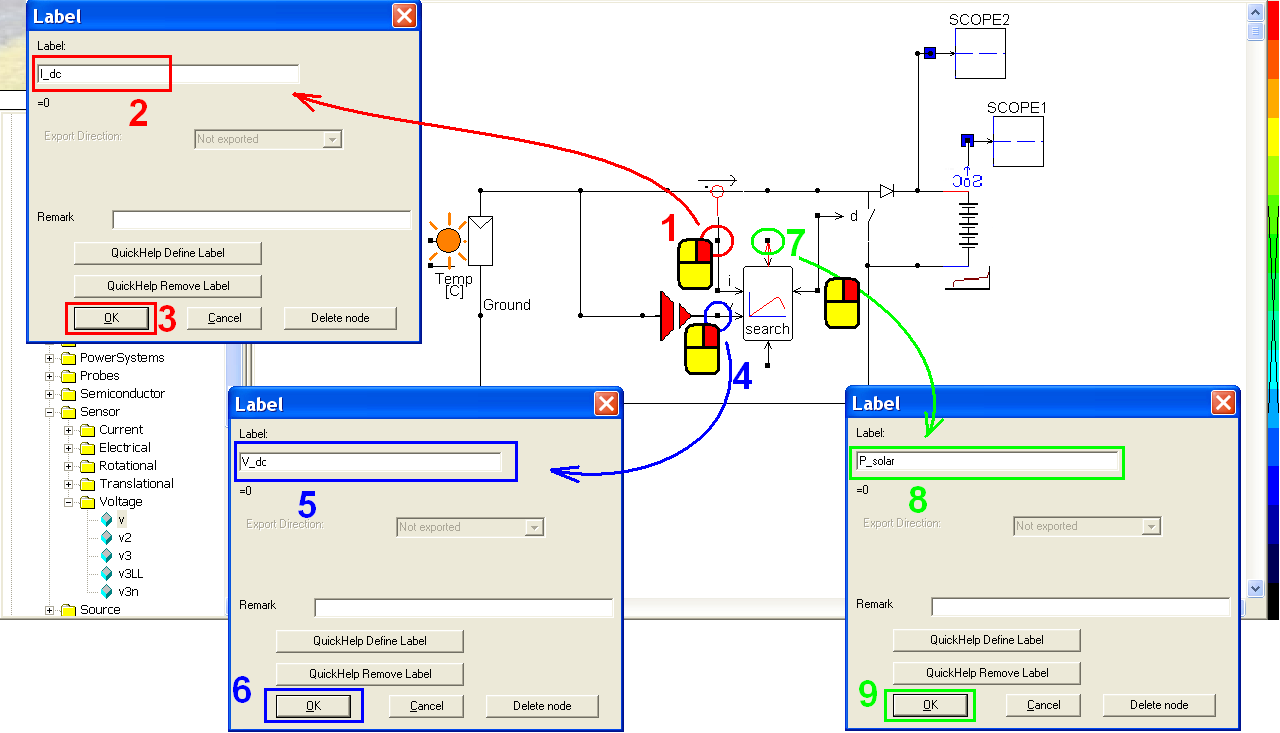Left-click Components/Blocks/Source/UPDOWN2 (step 1) and release the mouse. Left-click on the workscreen to put the updown above the solar cell. Left-click on the updown (step 2) to modify its parameters: Name = ESUM (step 3), Y0 = 1000, Ymin = 0, Ymax = 1000, Ystep = 100 (step 4). Click OK to save the setting (step 5).Connect the updown and the first input of the solar cell (step 1). Click the scope iconin the experience bar (step 2) to put a scope below the solar cell. Left-click the right-bottom corner of the scope and hold down the mouse to enlarge this scope (step 3).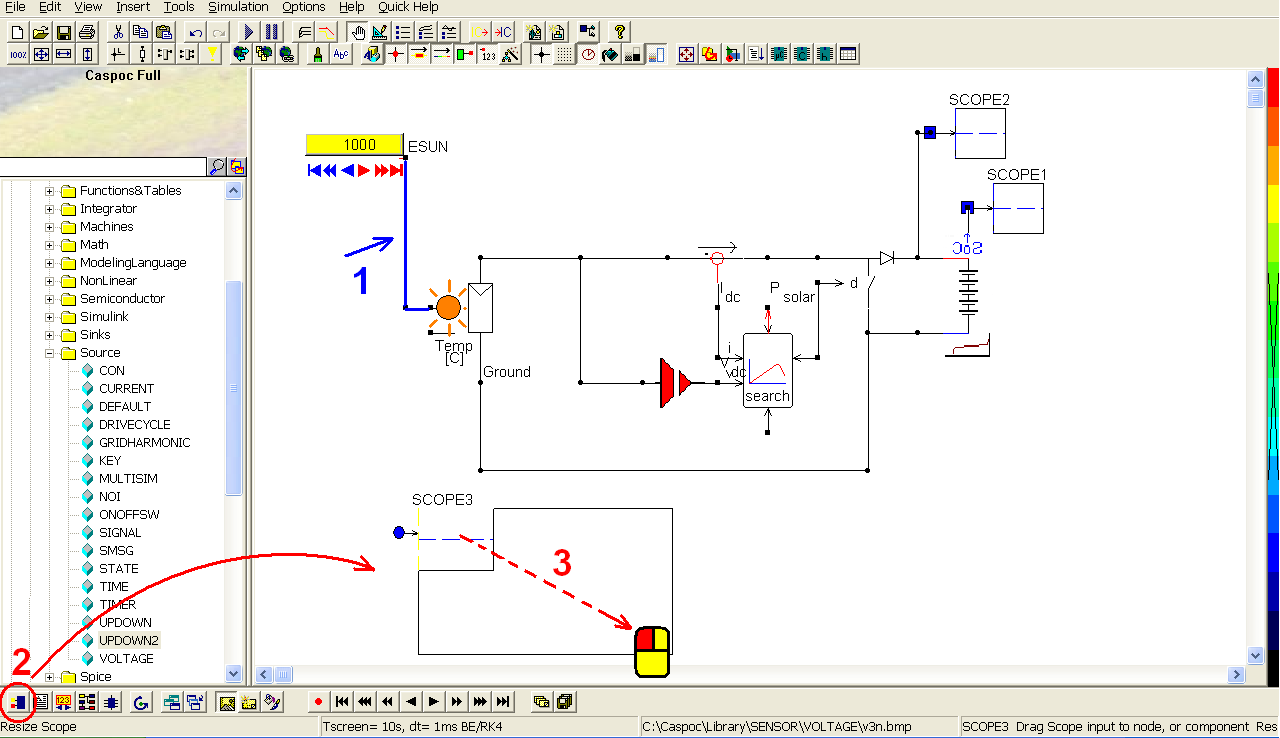Connect the first and third input traces of the third scope, similarly connect the second and fourth (step 1). To read the value of the current sensor namely I_dc (step 2), right-click on the square dot of the second and fourth input traces of scope 3 (step 3). In the pop-window, enter label = I_dc (step 4) and click OK to save the setting (step 5). To read the value of the voltage sensor namely V_dc (step 6), right-click on the square dot of the first and third input traces of scope 3 (step 7). In the pop-window, enter label = V_dc (step 8) and click OK to save the setting (step 9).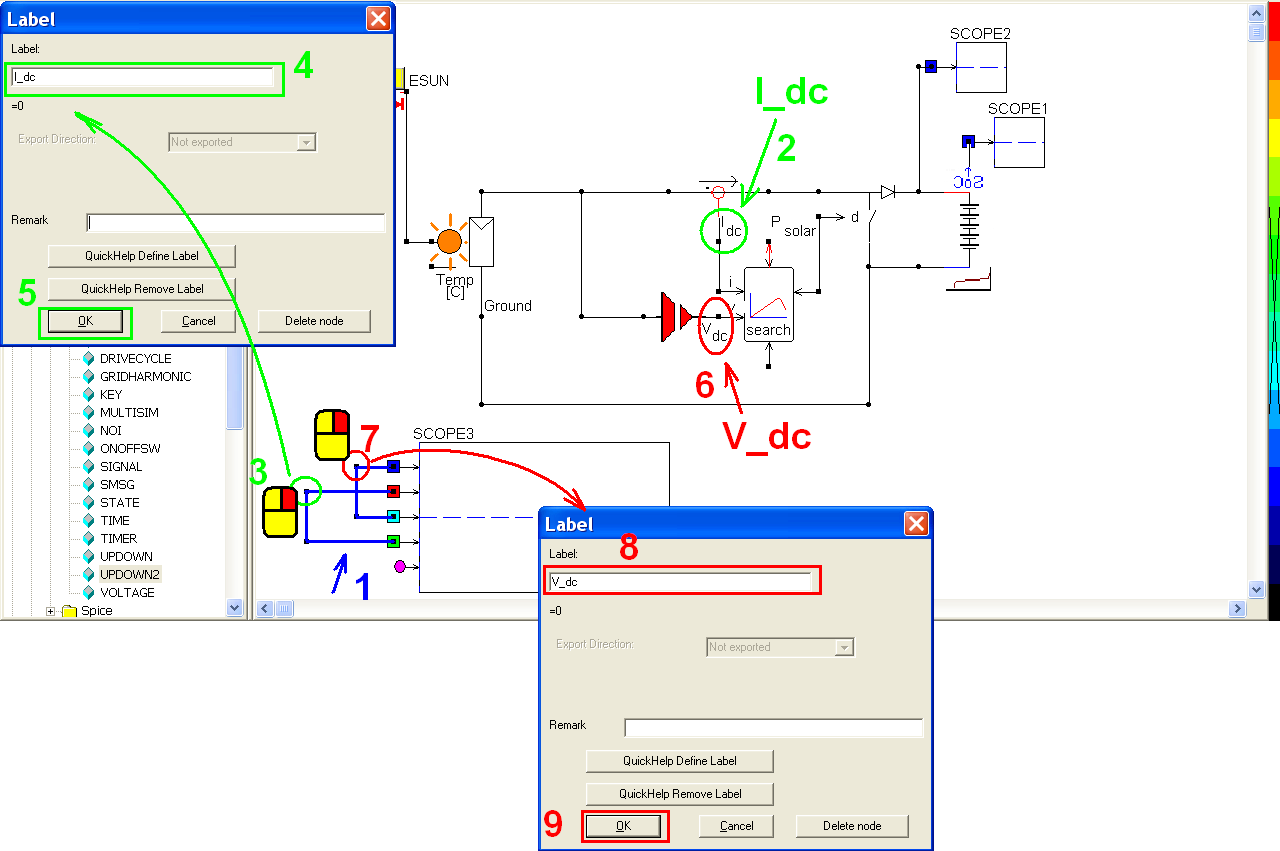Right-click on the third scope (step 1) and select View/XY Writer (step 2).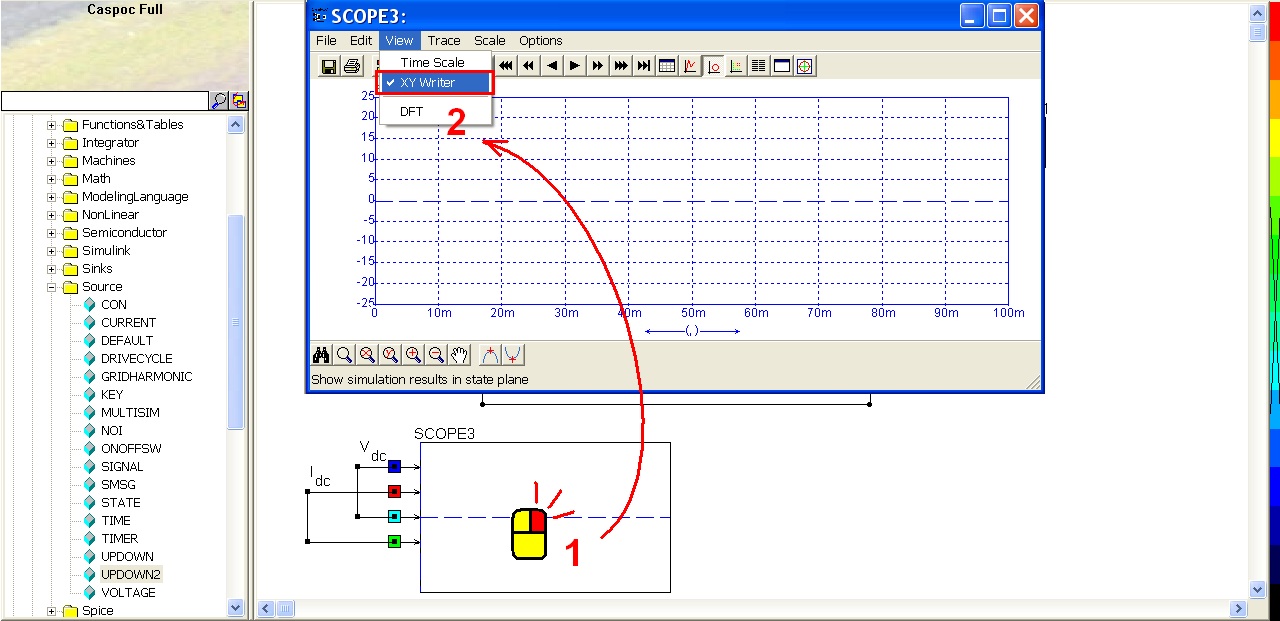Go to Trace/Trace2 (step 1). In the pop-window, select Appearance to Arrow (step 2) and click OK to save the setting (step 3).Click the scope iconin the experience bar (step 1) to put a scope in the right side of the third scope. Left-click the right-bottom corner of the scope and hold down the mouse to enlarge this scope (step 2).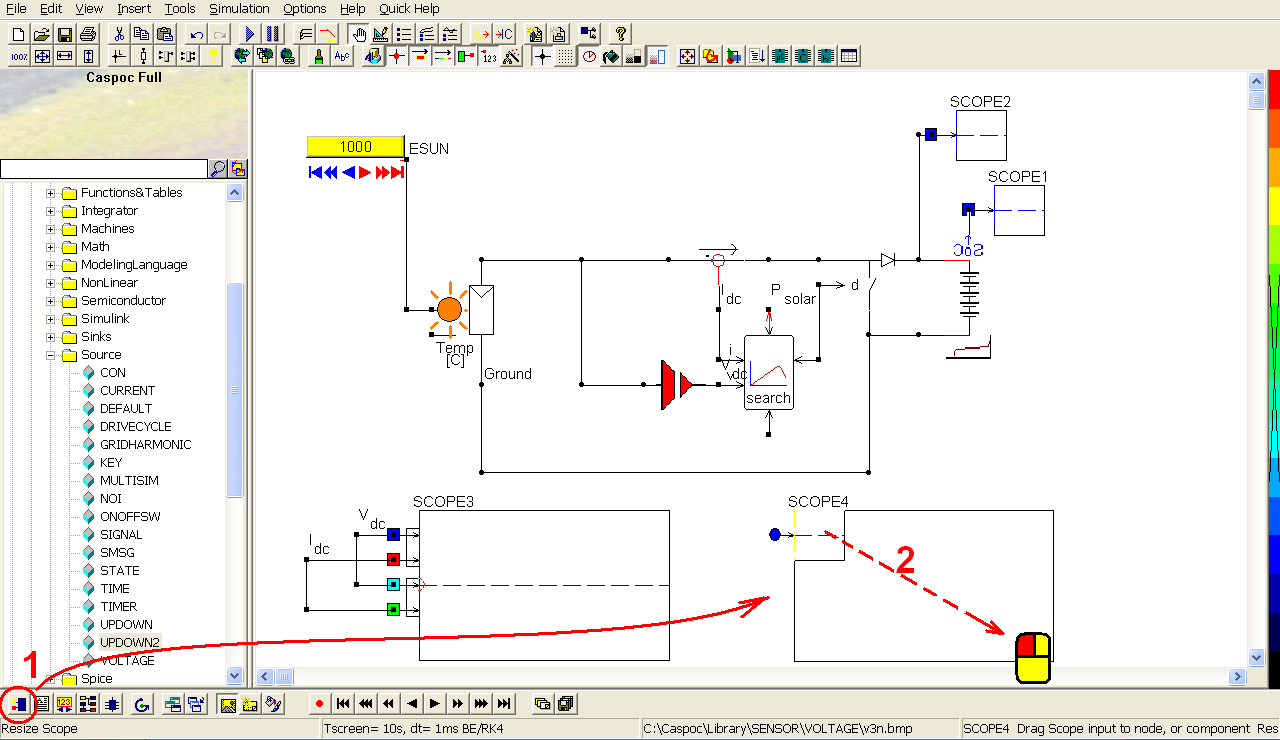To get the value of the solar power (measured on MPP searcher) (step 1), right-click on the first blue input trace of scope 4 and draw to extend it. Right-click on the extended input trace (square dot) to modify its parameter (step 2). In the pop-window, enter label = P_solar (step 3) and click OK to save the setting (step 4).Click the short-cut of simulation parameter(step 1). In the pop-window, select Euler for the Numerical Integration Method (step 2), Tscreen = 2, dt = 1m and select Wait after Screen (step 3). Then click OK to save the setting (step 4).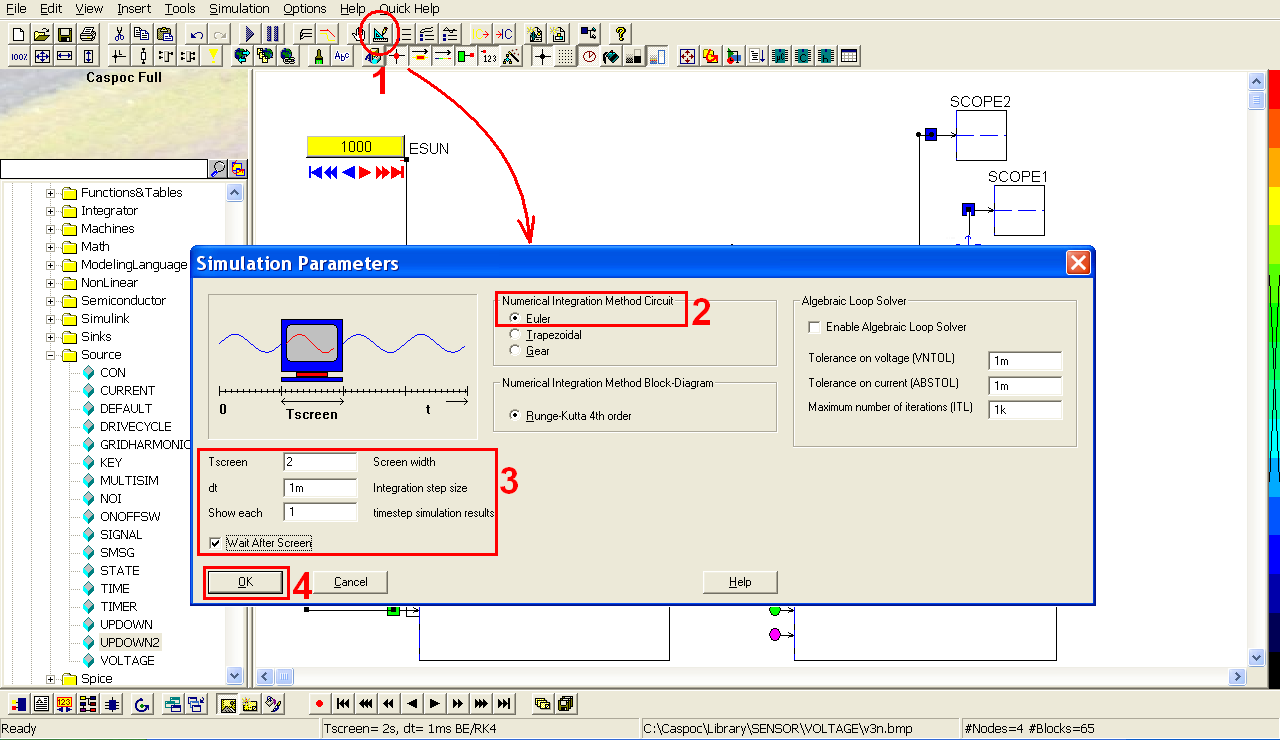Click the short-cut of start simulation(step 1). Right-click on the third scope (step 2) to see the details of the simulation result. In the pop-window, click the shortcut of listbox(step 3) to enable the numeric display of simulation result (step 4). Click Right or Left key to find the value change as time goes by (step 5). Right-click on the fourth scope (step 6) to see the details of the smulation reult. In the pop-window, click the shortcut of listbox(step 7) to enable the numeric display of simulation result (step 8). Click Right or Left key to find the value change as time goes by (step 9).Click the short-cut of simulation parameter(step 1). In the pop-window, unselect Wait After Screen (step 1) and then click OK to save the setting (step3).Click the short-cut of start simulation(step 1). Click the arrows of updown component to increase or decrease the sunlight density (step 2). Then we will find that the V-I characteristic curve in scope 4 (step 3). As well as the power, the power value also decrease or arise (step 4) according to the sunglight density (step 4).